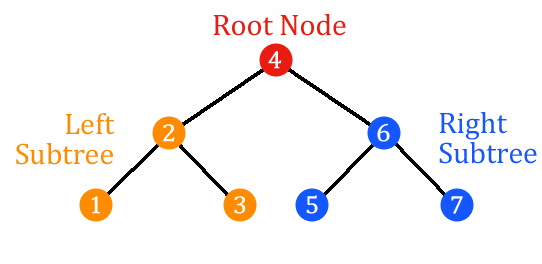# Postorder Traversal

Postorder traversal is a depth-first search algorithm for a binary search tree that first traverses the left subtree, then the right subtree, and then the root. Its primary use is deleting the tree.

## Algorithm

The postorder algorithm can be described as follows:

``````Function Postorder(tree)
return Postorder(left-subtree) + Postorder(right-subtree) + root
``````

## Example

For the following binary search tree:Postorder traversal provides the nodes in the following order: `1`, `3`, `2`, `5`, `7`, `6`, `4`.